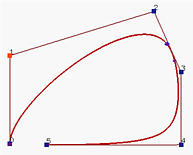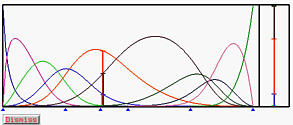# B-spline/NURBS Curves: Knot InsertionThe meaning of knot insertion is adding a new knot into the existing knot vector without changing the shape of the curve. This new knot can be equal to an existing one and in this case the multiplicity of that knot is increased by one. Because of the fundamental equality m = n + p + 1, after adding a new knot, the value of m is increased by one, and, consequently, either the number of control points or the degree of the curve must also be increased by one. Changing the degree of the curve will change the shape of the curve globally and will not be considered. Therefore, inserting a new knot causes a new control point to be added. In fact, some existing control points are removed and replaced with new ones by corner cutting.

Although knot insertion looks not very interesting, it is one of the most important algorithms for B-spline and NURBS curves since many other useful algorithms will be based on knot insertion. Therefore, we shall start with knot insertion algorithms. In the following, we shall present an algorithm for inserting a single knot.

The left figure below shows a clamped B-spline curve of degree 4 with uniformly spaced knots. The right figure shows the result after a new knot u = 0.5 is inserted. As you can see, the shape of the curve does not change; however, the defining control polyline is changed. In fact, four new control points (in red) replace the original control points p4, p5 and p6, and three line segments (in yellow) cut the corners at p4, p5 and p6.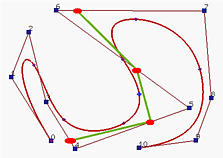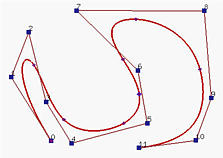## Inserting a Single Knot

Given a set of n+1 control points p0, p1, ..., pn, a knot vector of m+1 knots U = { u0, u1, ..., um } and a degree p, we want to insert a new knot t into the knot vector without changing the shape of the B-spline curve.

Suppose the new knot t lies in knot span [uk, uk+1). From the strong convex hull property, p(t) lies in the convex hull defined by control points pk, pk-1, ..., pk-p and all other basis functions are zero. Thus, the knot insertion computation can be restricted on control points pk, pk-1, ..., pk-p. The way of inserting t is to find p new control points qk on leg pk-1pk, qk-1 on leg pk-2pk-1, ..., and qk-p+1 on leg pk-ppk-p+1 such that the old polyline between pk-p and pk (in black below) is replaced by pk-pqk-p+1...qkpk (in orange below) by cutting the corners at pk-p+1, ..., pk-1. All other control points are unchanged. Note that p-1 control points of the original control polyline are removed and replaced with p new control points.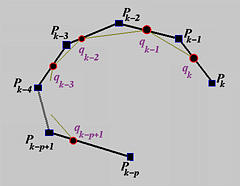Fortunately, the positions of new control points qi's are easy to compute. The formula for computing the new control point qi on leg pi-1pi is the following: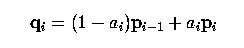where the ratio ai is defined below: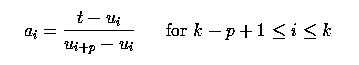In summary, to insert a new knot t, we first find the knot span [uk, uk+1) that contains this new knot. With k available, p new control points qk-p+1, ..., qk are computed with the above formula. Finally, the original control polyline between pk-p and pk is replaced with the new polyline defined by pk-p, qk-p+1, qk-p+2, ..., qk-1, qk and pk. Note that after inserting a new knot, the knot vector becomes u0, u1, ..., uk, t, uk+1, ..., and um. If the new knot t is equal to uk, the multiplicity of uk is increased by one.

The above computation scheme can be illustrated with the following diagram. First, the affected control points are listed on the left column. Then, the computed new control points are listed on the second column. Note that the number of new control points is one less than the affected control points. The computation of new control point qi, where k-p+1 <= i <= k, requires two original control points pi-1 and pi with coefficients 1-ai and ai, respectively. After the computation, we shall use the points surrounded by the blue dotted line to replace those not in the region. All unaffected control points are preserved. Therefore, the original set of control points pk-p, pk-p+1, ..., pk-1, pk is replaced with pk-p, qk-p+1, ..., qk-1, pk.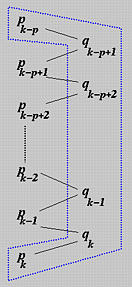Let us take a look at a geometric interpretation of ai. From its definition, ai is ratio of dividing interval [ui, ui+p) by the value of t as shown below: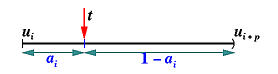There are k ai's, each of which covers p knot spans (i.e., [ui, ui+p)). If we stack these intervals together and align at the value of t, we have the following diagram: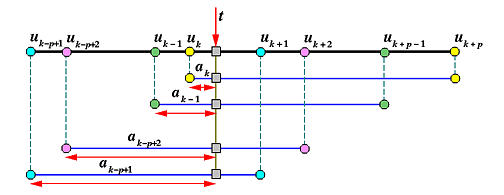Therefore, the position of t divides knot spans [uk, uk+p), [uk-1, uk+p-1), ..., [uk-p+1, uk+1) into ratios ak, ak-1, ...,, ak-p+1, which, in turn, provide the same ratios for dividing legs pkpk-1, pk-1pk-2, ... pk-ppk-p+1. ,

### Example 1: Inserting a Knot in a Knot Span

Suppose we have a degree 3 B-spline curve with a knot vector as follows:

 u0 to u3 u4 u5 u6 u7 u8 to u11 0 0.2 0.4 0.6 0.8 1

We want to insert a new knot t = 0.5. Since t = 0.5 lies in knot span [u5,u6), the affected control points are p5, p4, p3 and p2. To determine the three new control points q5, q4 and q3, we need to compute a5, a4 and a3 as follows:

a5 = (t - u5) / (u8 - u5) = (0.5 - 0.4) / ( 1 - 0.4) = 1/6
a4 = (t - u4) / (u7 - u4) = (0.5 - 0.2) / ( 0.8 - 0.2) = 1/2
a3 = (t - u3) / (u6 - u3) = (0.5 - 0) / ( 0.6 - 0) = 5/6
Therefore, the three new control points are
q5 = (1 - 1/6)p4 + (1/6)p5
q4 = (1 - 1/2)p3 + (1/2)p4
q3 = (1 - 5/6)p2 + (5/6)p3
The new control polyline becomes p0, p1, p2, q3, q4, q5, p5, ...., and the new knot vector is

 u0 to u3 u4 u5 u6 u7 u8 u9 to u12 0 0.2 0.4 0.5 0.6 0.8 1

Since the original B-spline curve has p = 3 and m = 11, we have n = m - p - 1 = 11 - 3 - 1 = 7 and hence 8 control points. The following is a B-spline curve satisfying this condition and its basis functions: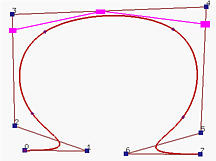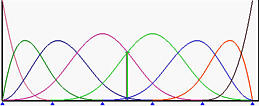The three blue rectangles are q3, q4 and q5. After inserting t = 0.5, the corners at p3 and p4 are cut, yielding the following B-spline curve and its basis functions. Note that the shape of the curve does not change: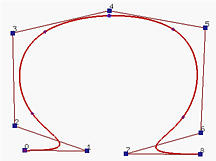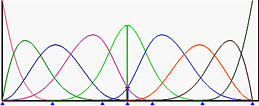The following diagram shows the relationship between the old and new control points. Note that the new set of control points contains p0, p1, p2, q3, q4, q5, p5, p6 and p7.The relationship among aj's, uj's, and t are shown below: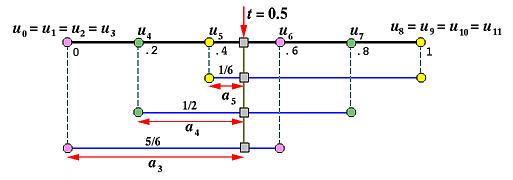### Example 2: Inserting a Knot at an Existing Simple Knot

Suppose we have a knot vector as follows:

 u0 to u4 u5 u6 u7 u8 u9 u10 u11 u12 to u16 0 0.125 0.25 0.375 0.5 0.625 0.75 0.875 1

We want to insert a new knot t = 0.5, which is equal to an existing one (i.e., t = u8 = 0.5). The following is a B-spline curve of degree 4 and its basis functions before the new knot t is inserted.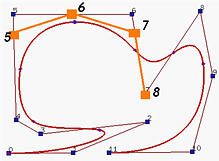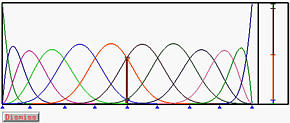Since t is in [u8,u9), the affected control points are p8, p7, p6, p5 and p4. The coefficients are computed as follows:

a8 = (t - u8) / (u12 - u8) = (0.5 - 0.5) / (1 - 0.875) = 0
a7 = (t - u7) / (u11 - u7) = (0.5 - 0.375) / (0.875 - 0.375) = 1/4
a6 = (t - u6) / (u10 - u6) = (0.5 - 0.25) / (0.75 - 0.25) = 1/2
a5 = (t - u5) / (u9 - u5) = (0.5 - 0.125) / (0.625 - 0.125) = 3/4
The new control points are
q8 = (1 - 0)p7 + 0p8
q7 = (1 - 1/4)p6 + (1/4)p7
q6 = (1 - 1/2)p5 + (1/2)p6
q5 = (1 - 3/4)p4 + (3/4)p5
The new control point q8 is equal to the original control point p7. In fact, if t is equal to a knot, say uk, then
ak = (t - uk) / (uk+p - uk) = 0
Consequently, we have
qk = (1 - 0)pk-1 + 0pk = pk-1
That is, if the new knot t to be inserted is equal to an existing simple knot uk, then qk, the last new control point, is equal to pk-1. The following diagram is the computation scheme: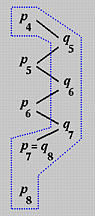The figure shown at the beginning of this example shows the new control points and the new control polyline in orange, Please note that the new control points are p0, p1, p2, p3, p4, q5, q6, q7, q8 = p7, p8, p9, p10 and p11. The curve and its basis functions after new knot t = 0.5 inserted is shown below.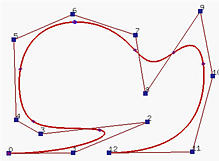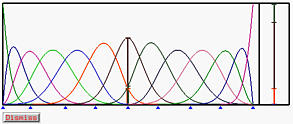The relationship among aj's, uj's, and t are shown below: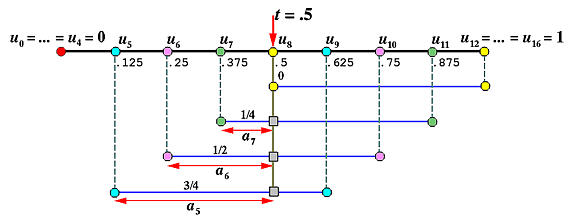### Example 3: Inserting a Knot at an Existing Multiple Knot

What if the new knot t is inserted at a multiple knot? Suppose t is inserted at knot uk of multiplicity s. Hence, we have s consecutive equal knots: uk = uk-1 = uk-2 = .... = uk-s+1 and uk-s+1 being not equal to uk-s. In the computation of coefficients ak, ...., ak-p+1, we have the following:

ak = (t - uk) / (uk+p - uk) = (uk - uk) / (uk+p - uk) = 0
ak-1 = (t - uk-1) / (uk+p-1 - uk-1) = (uk - uk-1) / (uk+p-1 - uk-1) = 0
..........
ak-s+1 = (t - uk-s+1) / (uk-s+1+p - uk-s+1) = (uk - uk-s+1) / = 0
Hence, coefficients ak, ...., ak-p+1 are all zero and consequently we have
qk = (1 - ak)pk-1 + akpk = pk-1
qk-1 = (1 - ak-1)pk-2 + ak-1pk-1 = pk-2
..........
qk-s = (1 - ak-s+1) pk-s + ak-s+1pk-s = pk-s
This shows that if the new knot t is inserted at a knot uk of multiplicity s, then the last s new control points, qk, qk-1, ..., qk-s+1 are equal to the original control points pk-1, pk-2, ..., pk-s. If s = 1 (i.e., simple knot), qk is equal to pk-1, which is exactly what has been discussed in Example 2. If s = 0 (i.e., t is not a knot), then all control points from pk-p to pk are involved. This is the case of Example 1. The following diagram is the computation scheme: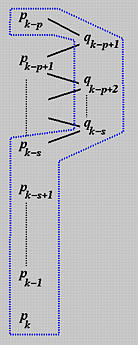## Knot Insertion for NURBS Curves

The above discussion of knot insertion is for B-spline curves. Since NURBS curves are the projections of 4D B-spline curves to 3D, knot insertion for NURBS curves is easy. Note that the above discussion and computation does not require the control points being in the three dimensional space. Therefore, knot insertion for NURBS curves is done in three steps: (1) converting the given NURBS curve in 3D to a B-spline curve in 4D, (2) performing knot insertion to this four dimensional B-spline curve, and (3) projecting the new set of control points back to 3D to form the the new set of control points for the given NURBS curve after the required knot has been inserted.

Suppose we have n + 1 control points p0, p1, ..., pn with associated weights w0, w1, ..., wn, a knot vector U and a degree p. Let pi = (xi, yi, zi). Then, control points Pi = ( wixi, wiyi, wizi, wi ), 0 <= i <= n, and knot vector U define a four dimensional B-spline of degree p. We can insert a knot t to this four dimensional B-spline yielding a new set of control points Qi = ( Xi, Yi, Zi, Wi ), 0 <= i <= n. Projecting these control points back to the three-dimensional space by dividing the first three components with the fourth one yields the new set of control points of the given NURBS curve.

Let us take a look at an example. Suppose we have 9 knots

 u0 to u3 u4 u5 to u8 0 0.5 1

and a NURBS curve of degree 3 defined by the following 5 control points in the xy-plane:

 x y w p0 -70 -76 1 p1 -70 75 0.5 p2 74 75 4 p3 74 -77 5 p4 -40 -76 1

The following shows the curve and its basis functions.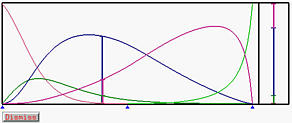Let us insert a new knot t = 0.4. Since t is in knot span [u3, u4) and the degree of the NURBS curve is 3, the affected control points are p3, p2, p1 and p0. Since this is a NURBS curve, we shall use homogeneous coordinates by multiplying all control points with their corresponding weights. Call these new control points Pi:

 x y w P0 -70 -76 1 P1 -35 37.5 0.5 P2 296 300 4 P3 370 -385 5

Note that since P4 is not affected, it is not computed in the above table. Then, we shall compute a3, a2 and a1 as follows:

a3 = (t - u3)/ (u6 - u3) = (0.4 - 0)/(1 - 0) = 0.4
a2 = (t - u2)/ (u5 - u2) = (0.4 - 0)/(1 - 0) = 0.4
a1 = (t - u1)/ (u4 - u1) = (0.4 - 0)/(0.5 - 0) = 0.8
The new control points Q3, Q2 and Q1 are:
Q3 = (1 - a3)P2 + a3P3 = ( 325.6, 26, 4.4 )
Q2 = (1 - a2)P1 + a2P2 = ( 97.4, 142.5, 1.9 )
Q1 = (1 - a1)P0 + a1P1 = ( -42, 14.8, 0.6 )
Projecting these three four dimensional control points by dividing the first two components with the third (the weight), we have
q3 = ( 74, 5.9 ) with weight 4.4
q2 = ( 51.3, 75 ) with weight 1.9
q1 = ( -70, 24.6 ) with weight 0.6

The following is the resulting NURBS curve and its basis functions: